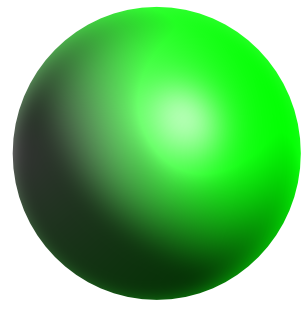Volume of a Sphere

## Volume of a Sphere

The volume of a sphere is given by:

V = frac(4)(3)pir^3## Example 1

What is the volume of a sphere of diameter 6 cm? Give your answer to 3 significant figures.

 For a sphere: Volume = frac(4)(3)pir^3 Substitute =frac(4)(3)pi(6)^3 = 904.778

 Cylinder: Volume = pir^2l Sphere: Volume = frac(4)(3)pir^3 Both equal pir^2l = frac(4)(3)pir^3 Divide both sides by pi r^2l = frac(4)(3)r^3 Divide both sides by r^2 l = frac(4)(3)r Substitute l = frac(4)(3)(9) = 12